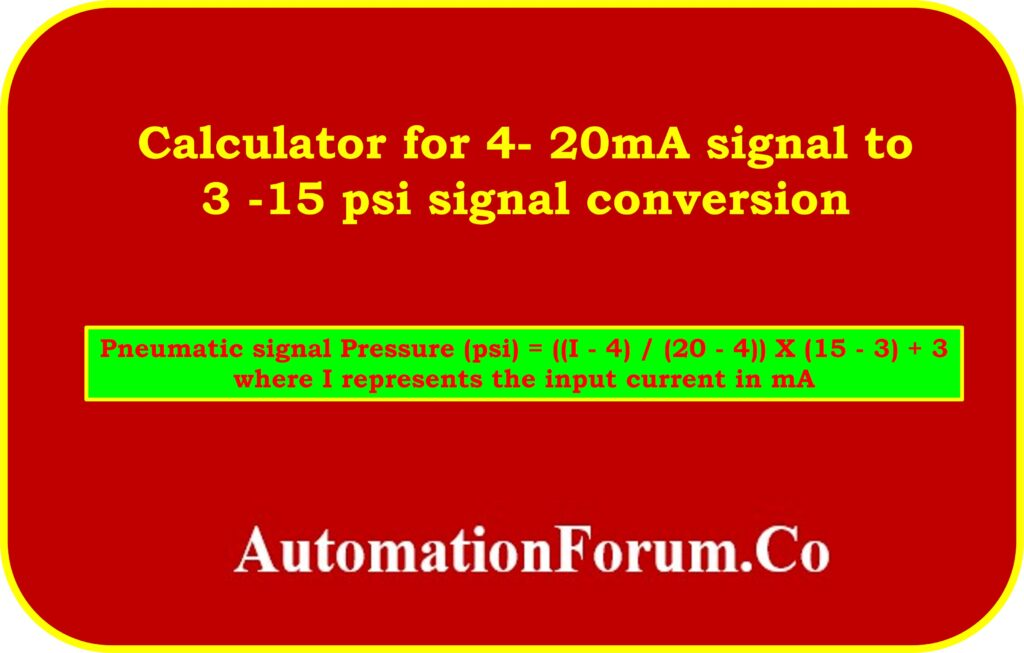# Calculator for 4- 20mA signal to 3-15 psi signal conversionAn instrumentation calculator for converting a standard 4-20mA signal to a 3-15 psi signal is a tool used in industrial automation and control systems. This conversion is commonly required in process control applications where different types of instruments are used to measure and control variables such as pressure, level, or flow.

The 4-20mA signal is widely used in industrial instrumentation due to its robustness and ability to transmit analog data over long distances without significant loss or interference. On the other hand, the 3-15 psi signal is a common range for pneumatic instruments, where pressure is used to transmit and control signals.

## Formula for the signal conversion

To convert the 4-20mA signal to a 3-15 psi signal, a linear conversion can be applied using the following formula:

Pressure (psi) = ((I – 4) / (20 – 4)) X (15 – 3) + 3

Where, I represent the input current in mA.

This formula calculates the corresponding pneumatic pressure signal value within the 3-15 psi range based on the input current.

## Break down the formula:

• Subtracting 4 from the input current (I – 4) shifts the range from 4-20mA to 0-16mA.
• Dividing the shifted current value by the range of the input signal (20 – 4) normalizes the value between 0 and 1.
• Multiplying the normalized value by the range of the output signal (15 – 3) scales the value to the desired range.
• Finally, adding the minimum value of the output signal (3) gives the converted pressure value in psi.

## Example calculation

For example, if the input current is 12.20mA, we can substitute it into the formula:

Pressure (psi) = ((12.2 – 4) / (20 – 4)) * (15 – 3) + 3

Pressure (psi) = (8.2 / 16) * 12 + 3

Pressure (psi) = 0.513 * 12 + 3

Pressure (psi) = 6.15 + 3

Pressure (psi) = 9.15 psi

Therefore, for an input current of 12.20mA, the corresponding pressure value would be 9.15 psi.

This instrumentation calculator simplifies the conversion process by automating the calculation for various input current values, allowing engineers and technicians to easily determine the corresponding pressure values in psi. This conversion enables seamless integration between instruments using different signal types and ensures accurate measurement and control in industrial processes.

## Signal calculator

The following instrumentation calculator will convert standard 4- 20mA signals to 3-15 psi signals.

Scroll to Top